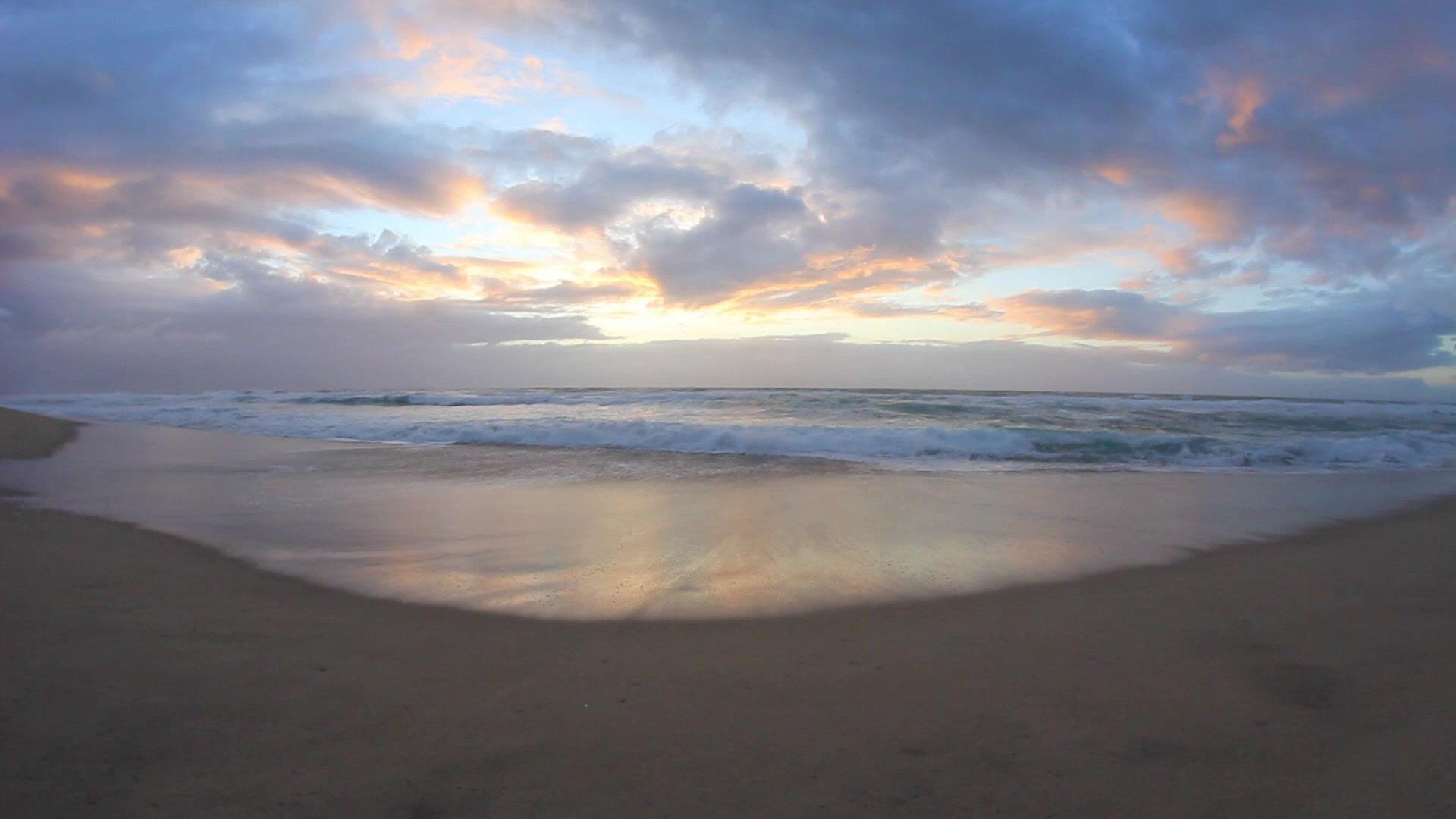top of pageDwindle's Dream Fishing Charters

2023 Rates Breakdown

Rates Include All Fees Except Any Totally Optional Gratuities

​"Arrive As Our Client Depart As Our Friend"

Wow! What A Boat!

Charters Offered 7 Days A Week For Groups Of Up To 12+ Persons

Please Note 5 and 6 Hour Afternoon Charters Are Minimum Of 1 To 6 PeopleHalf Day 5 Hour Charter

TOTAL GROUP COST:

1 TO 5 Persons AM: \$850.00 *** 1 TO 6 Persons PM: \$970.00

Family Rate: Minimum Boat Charge \$850.00 AM \$1,080.00 PM

Children 17 & Under Free

APPROXIMATE PER PERSON BREAK DOWN:

1 Person = \$850.00 (Afternoon \$970.00)

2 Persons = \$425.00 Each (Afternoon \$970.00 = \$485.00)
3 Persons = \$283.34 Each (Afternoon \$970.00 = \$323.34)
4 Persons = \$212.50 Each (Afternoon \$970.00 = \$242.50)
5 Persons = \$170.00 Each (Afternoon \$970.00 = \$194.00)
6 Persons \$970.00 = \$161.67 Each (Afternoon \$970.00= \$161.67 Each)

7 Persons \$1,090.00 = \$155.71

8 Persons \$1,210.00 = \$151.25 Each

9 Persons \$1,330.00 = \$147.78 Each

10 Persons \$1,450.00 = \$145.00 Each

11 Persons \$1,570.00 = \$142.73 Each

​12 Persons \$1,690.00 = \$140.83 Each

Half Day 6 Hour Charter

TOTAL GROUP COST:

1 TO 5 Persons AM: \$960.00 *** 1 TO 6 Persons PM: \$1,080.00

Family Rate: Minimum Boat Charge \$960.00 AM \$1,080.00 PM

Children 17 & Under Free

APPROXIMATE PER PERSON BREAK DOWN:

1 Person = \$960.00
(Afterno
on = \$1,080.00)

2 Persons = \$480.00 Each
(Afternoon \$1,080.00 = \$540.00 Each)

3 Persons = \$320.00 Each
(Afternoon \$1,080.00 = \$360.00 Each)

4 Persons = \$240.00 Each
(Afternoon \$1,080.00 = \$270.00 Each)

5 Persons = \$192.00 Each
(Afternoon \$1,080.00 = \$216.00 Each)

6 Persons \$1080.00 = \$180.00 Each
(
Afternoon \$1,080.00 = \$180.00 Each)

​7 Persons \$1,200.00 = \$171.43 Each

​8 Persons \$1,320.00 = \$165.00 Each

9 Persons \$1,440.00 = \$160.00 Each

10 Persons \$1,560.00 = \$156.00 Each

11 Persons \$1,680.00 = \$152.72 Each

​12 Persons \$1,800.00 = \$150.00 Each​

Full Day 8 Hour Charter

TOTAL GROUP COST:

1 to 5 Persons \$1,200.00

Family Rate: Minimum Boat Charge \$1,200.00
Children 17 & Under Free

APPROXIMATE PER PERSON BREAK DOWN:

1 Person = \$1,200.00
2 Persons = \$600.00 Each
3 Persons = \$400.00 Each
4 Persons = \$300.00 Each
5 Persons = \$240.00 Each

6 Persons \$1,330.00 = \$221.67 Each

7 Persons \$1,460.00 = \$208.57 Each

8 Persons \$1,590.00 = \$198.75 Each

9 Persons \$1,720.00 = \$191.11 Each

10 Persons \$1,850.00 = \$185.00 Each

11 Persons \$1,980.00 = \$180.00 Each

12 Persons \$2,110.00 = \$175.83 Each​

Full Day 10 Hour Charter

TOTAL GROUP COST:

1 to 5 Persons \$1,440.00

Family Rate: Minimum Boat Charge \$1,440.00
Children 17 & Under Free

APPROXIMATE PER PERSON BREAK DOWN:

1 Person = \$1,440.00
2 Per
sons = \$720.00 Each
3 Persons = \$480.00 Each

4 Persons = \$360.000 Each
5 Persons = \$288.00 Each

6 Persons \$1,590.00 = \$265.00 Each
7 Persons \$1,740.00 = \$248.57 Each

8 Persons \$1,890.00 = \$236.25 Each
9 Persons \$2,040.00 = \$226.67 Each
10 Persons \$2,190.00 = \$219.00 Each
11 Persons \$2,340.00 = \$212.72 Each

12 Persons \$2,490.00 = \$207.50 Each

Full Day 8 Hour "Offshore Charter"

TOTAL GROUP COST:

1 to 5 Persons \$1,500.00

Family Rate: Minimum Boat Charge \$1,500.00
Children 17 & Under Free

APPROXIMATE PER PERSON BREAK DOWN:

1 Person = \$1,500.00
2 Persons = \$750.00 Each
3 Persons = \$500.00 Each
4 Persons = \$375.00 Each
5 Persons = \$300.00 Each

6 Persons \$1,650.00 = \$275.00 Each

7 Persons \$1,800.00 = \$257.14 Each

8 Persons \$1,950.00 = \$243.75 Each

9 Persons \$2,100.00 = \$233.33 Each

10 Persons \$2,250.00 = \$225.00 Each

11 Persons \$2,400.00 = \$218.18 Each

12 Persons \$2,550.00 = \$212.50 Each

Full Day 10 Hour "Offshore Charter"

TOTAL GROUP COST:

1 to 5 Persons \$1,750.00

Family Rate: Minimum Boat Charge \$1,750.00
Children 17 & Under Free

APPROXIMATE PER PERSON BREAK DOWN:

1 Person = \$1,750.00
2 Persons = \$875.00 Each
3 Persons = \$583.33 Each
4 Persons = \$437.50 Each
5 Persons = \$350.00 Each

6 Persons \$1,900.00 = \$316.67 Each

7 Persons \$2,050.00 = \$292.86 Each

8 Persons \$2,200.00 = \$275.00 Each

9 Persons \$2,350.00 = \$261.11 Each

10 Persons \$2,500.00 = \$250.00 Each

11 Persons \$2,650.00 = \$240.91 Each

12 Persons \$2,800.00 = \$233.33 Each

Boat Tour: \$550.00   Funeral At Sea: \$450.00​

Additional Hours May Be Arranged at \$130.00 Per Hour (Per Group)

Page Maintained by Your Captain Rick Dwinnell

Dwinnell's Computer Services

bottom of page